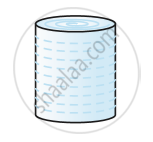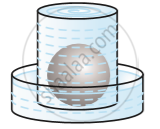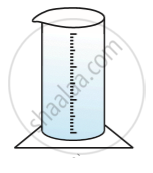# Volume of a Sphere

#### notes

Now, let us see how to go about measuring the volume of a sphere.
First, take two or three spheres of different radii, and a container big enough to be able to put each of the spheres into it, one at a time. Also, take a large trough in which you can place the container. Then, fill the container up to the brim with water  in following fig.Now, carefully place one of the spheres in the container. Some of the water from the container will over flow into the trough in which it is kept in following fig.Carefully pour out the water from the trough into a measuring cylinder (i.e., a graduated cylindrical jar) and measure the water over flowed in following fig.Suppose the radius of the immersed sphere is r .
Volume of a Sphere = 4/3 πr^3
where r is the radius of the sphere.
So, Volume of a Hemisphere = 2/3 πr^3
where r is the radius of the hemisphere.

If you would like to contribute notes or other learning material, please submit them using the button below.

### Shaalaa.com

Volume of Sphere [00:05:16]
S
0%